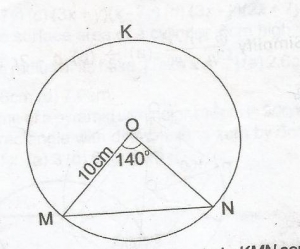In the diagram, MN is a chord of a circle KMN centre O and radius 10cm. If < MON = 140o, find, correct to the nearest cm, the length of the chord MN.

A. 19cm
B. 18cm
C. 17cm
D. 12cm

Correct Answer: Option A

A. 19cm

Explanation
From the diagram

sin 70° = x/10

x = 10sin 70°

= 9.3969

Hence, length of chord MN = 2x

= 2 x 9.3969

= 18.7938

= 19cm (nearest cm)